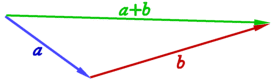Go to contentYou can get a better display of the maths by downloading special TeX fonts from jsMath. In the meantime, we will do the best we can with the fonts you have, but it may not be pretty and some equations may not be rendered correctly.

## Glossary

### union

The union of two sets A and B is the set containing all the elements of A and B.

Full Glossary List

## This question appears in the following syllabi:

SyllabusModuleSectionTopicExam Year
AQA A-Level (UK - Pre-2017)C4VectorsVector algebra-
AQA AS Maths 2017MechanicsVectorsVector Basics-
AQA AS/A2 Maths 2017MechanicsVectorsVector Basics-
CBSE XII (India)Vectors and 3-D GeometryVectorsTypes, equal, unit, parallel, collinear-
CCEA A-Level (NI)C4VectorsVector algebra-
CIE A-Level (UK)P1VectorsVector algebra-
Edexcel A-Level (UK - Pre-2017)C4VectorsVector algebra-
Edexcel AS Maths 2017Pure MathsVectorsVector Basics-
Edexcel AS/A2 Maths 2017Pure MathsVectorsVector Basics-
I.B. Higher Level4VectorsVector algebra-
I.B. Standard Level4VectorsVector algebra-
Methods (UK)M4VectorsVector algebra-
OCR A-Level (UK - Pre-2017)C4VectorsVector algebra-
OCR AS Maths 2017Pure MathsVectorsVector Basics-
OCR MEI AS Maths 2017Pure MathsVectorsVector Basics-
OCR-MEI A-Level (UK - Pre-2017)C4VectorsVector algebra-
Pre-Calculus (US)E1VectorsVector algebra-
Pre-U A-Level (UK)6VectorsVector algebra-